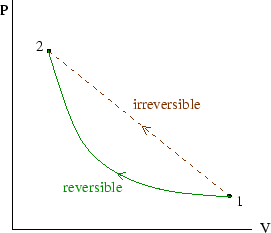Next: Heat engine examples Previous: Efficiency of ideal gas Carnot cycle

## Reversible processes

Imagine a cylinder, with a perfectly smooth piston, which contains gas. If you push with a force only just large enough to overcome the internal pressure, the volume will start to decrease slowly. Then if you decrease the force only slightly, the volume will start to increase. This is the hallmark of a reversible process: an infinitesimal change in the external conditions reverses the direction of the change. Heat flow is only reversible if the temperature difference between the bodies is infinitesimally small.

Reversible processes require the absence of friction or other hysteresis effects. They must also be carried out infinitesimally slowly. Otherwise pressure waves and finite temperature gradients will be set up in the system, and irreversible dissipation and heat flow will occur.Because reversible processes are very slow, the system is always very nearly in equilibrium at all times. In that case all its state variables are well defined and uniform, and the state of the system can be represented on a plot of, for instance, pressure versus volume. A finite reversible process passes through an infinite set of such states, and so can be drawn as a solid line on the plot. During an irreversible process the system is not in an equilibrium state, and so cannot be represented on the plot; an irreversible process is often drawn as a straight dotted line joining the initial and final equilibrium states. The work done during the process is not then equal to the area under the line, but will be greater than that for the corresponding reversible process.Next: Heat engine examples Previous: Efficiency of ideal gas Carnot cycle
Judith McGovern 2004-03-17